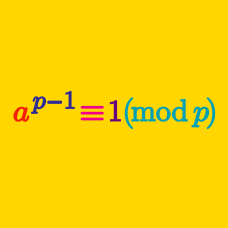Number Theory

Basic Applications of Modular Arithmetic: Level 2 Challenges

The number of students in a school is between 500 and 600. If we group them into groups of 12, 20, or 36 each, 7 students are always left over. How many students are in this school?

Find the remainder when $2016!-2015!$ is divided by $2017$.

You may use the fact that $2017$ is prime.

What is the remainder when ${7}^{88}$ is divided by $11?$

What is the remainder when $\Huge \color{#D61F06}{12}^{\color{#20A900}{34}^{\color{#3D99F6}{56}^{\color{#624F41}{78}}}}$ is divided by $\color{#302B94}{90}?$

What is the smallest positive integer which is a multiple of 7, yet it gives a remainder of 1 when divided by any of 2, 3, 4, 5, or 6?

×